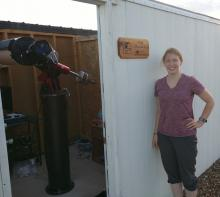# Iraf filter/detector info for use with ccdtime?

3 posts / 0 new
MeekaIraf filter/detector info for use with ccdtime?

I'm trying to get set up with Iraf/Pyraf since I need a more automated solution in the long term, my scope is producing more data than I can keep up with and still work full time. One of the functions available that I'm interested in is using ccdtime to get approximate exposure times for each filter to start from so I waste fewer exposures. I know it will also depend on the type of star, but I'll figure that out next.

My primary question is how to calculate the information needed for the filters and detector to store in the .dat file.  There is a file with the defaults for the NOAO scopes, and I tried using that as a starting point for getting my info set up.  A subset of that file is below, and I think I have the detector part sorted out, but I'm not sure how to handle the filters. My initial question is how to calculate the star counts, since a file comment mentions the current ones are for photons/second/stellar image for a 1m telescope at 1 airmass. I'm not sure how to scale that to fit my setup, I tried using the counts for each filter for a star (and the sky) from the M67 field taken with this ccd and scope, but the results from running ccdtime were ridiculously high in terms of the time needed to get a SNR of 100 for an 11 mag. star. Any suggestions on how to calculate the star count and extinction data for a setup other than the defaults?

Default file:

telescope = "0.9m"
aperture = 0.81
scale = 28.3
transmission = 1.0

# Filter data:
# Magnitude corresponding to photon count rate.
# Photons/second/stellar-image for a 1-m telescope at 1 airmass.
# Sky magnitudes per sq arc sec:
# sky = sky0 + sky1 * phase + sky2 * phase**2 (phase=0-14)

filter = "U"
extinction = 0.55
mag = 20
star = 8.0
sky0 = 22.0
sky1 = -0.2666
sky2 = -.00760

filter = "B"
extinction = 0.25
mag = 20
star = 34.5
sky0 = 22.7
sky1 = -0.0998
sky2 = -0.00953

filter = "V"
extinction = 0.14
mag = 20
star = 36.2
sky0 = 21.8
sky1 = -0.0153
sky2 = -0.00838

filter = "R"
extinction = 0.10
mag = 20
star = 36.8
sky0 = 20.9
sky1 = -0.0211
sky2 = -0.00364

filter = "I"
extinction = 0.05
mag = 20
star = 26.7
sky0 = 19.9
sky1 = -0.0086
sky2 = -0.00311

# Detector information:
# rdnoise in photons, dark rate in photons per second, pixel size in microns,
# and the effective DQE for each filter.

detector = "MOSAIC1_1"
rdnoise = 4.9
dark = 0.00102
pixsize = 15
U = 0.55
B = 0.84
V = 0.84
R = 0.84
I = 0.79
z = 0.77

My info for the detector/scope:

telescope = "M2"
aperture = 0.3175
scale = 97.2
transmission = 1.0

detector = "SBIG"
rdnoise = 15
dark = 0.00102
pixsize = 9
U = 0.50
B = 0.65
V = 0.84
R = 0.84
I = 0.65

HQAphoton flux

Hi Meeka,

http://topics.sirtf.com/Astro4410/EstimatingPhotons

You can also ask for help on iraf.net.

Arne

MeekaThanks Arne, this was exactly

Thanks Arne, this was exactly the kind of resource I was looking for. Now I think my results are looking accurate enough to test.